# Mechanical Properties Of Materials - 3

## 30 Questions MCQ Test Topicwise Question Bank for GATE Mechanical Engineering | Mechanical Properties Of Materials - 3

Description
This mock test of Mechanical Properties Of Materials - 3 for Mechanical Engineering helps you for every Mechanical Engineering entrance exam. This contains 30 Multiple Choice Questions for Mechanical Engineering Mechanical Properties Of Materials - 3 (mcq) to study with solutions a complete question bank. The solved questions answers in this Mechanical Properties Of Materials - 3 quiz give you a good mix of easy questions and tough questions. Mechanical Engineering students definitely take this Mechanical Properties Of Materials - 3 exercise for a better result in the exam. You can find other Mechanical Properties Of Materials - 3 extra questions, long questions & short questions for Mechanical Engineering on EduRev as well by searching above.
QUESTION: 1

Solution:
QUESTION: 2

Solution:
QUESTION: 3

### The following figure shows the stress-strain curve for tensile test on a mild steel specimen.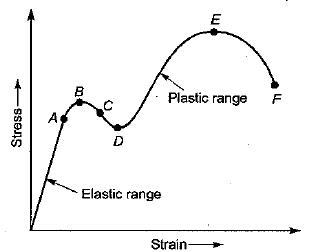Q. The upper yield point is represented by the point

Solution:
QUESTION: 4

Select the proper sequence
1. Proportional limit
2. Elastic limit
3. Yielding
4. Failure

Solution: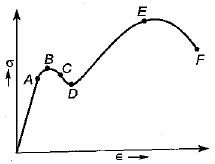A: Proportional limit
B : Elastic limit
C : UYP
D: LYP
E: UTS
F: Rupture point

QUESTION: 5

The stress-strain curve for glass during tensile test would exhibit

Solution:

Glass is a brittle material and it can’t withstand tension.

QUESTION: 6

The impact strength of a material is an index of its

Solution:
QUESTION: 7

Pick the odd one out

Solution:

Except endurance limit all are elastic properties of the material in tension. Endurance limit is the fatigue property and found out by reversible cyclic loading.

QUESTION: 8

What are the materials which show direction dependent properties?

Solution:

Anisotropy is the property in which material possess different properties in different directions.

QUESTION: 9

A material has identical properties in all directions, it is said to be

Solution:

Isotropy is the property in which material posses same properties any where within it.

QUESTION: 10

Creep is the gradual increase of

Solution:

Creep is that property by virtue of which a metal specimen undergoes additional deformation with the passage of time under sustained loading within elastic limit. It is permanent in nature and cannot be recovered after removal of load, hence is plastic in nature.

QUESTION: 11

Resilience is

Solution:

Resilience is the total strain energy stored in a given volume of a material within elastic limit. On removal of load this energy is released. In other words, it is the area under load deflection curve within elastic limit.

QUESTION: 12

The material in which large deformation is possible before the absolute failure or rupture is termed as

Solution:

Ductility is defined as the ability of the material to deform to a greater extent before the sign of crack, when it is subjected to tensile force. Ductile materials are those materials which deform plastically to a greater extent prior to fracture e.g. mild steel, copper and aluminium.

QUESTION: 13

What is the phenomenon of progressive extension of the material i.e. strain increasing with the time at a constant load, called?

Solution:

When metals are subjected to constant loads, metals tends to deform continuously. This continuous deformation of metals under constant loads is called as creep.

QUESTION: 14

The stress level, below which a material has a high probability of not failing under reversal of stress, is known as

Solution:

The stress which can be withstand for some specified number of cycles is the fatigue strength of material. The stress level which can be withstand for an infinite number of cycles, without failure is called endurance limit.

QUESTION: 15

The energy absorbed in a body, when it is strained within the elastic limits, is known as

Solution:

Resilience can be defined as the ability of the material to absorb energy when deformed elastically and to release this energy when unloaded.

QUESTION: 16

Toughness for mild steel under uniaxial tensile loading is given by the shaded portion of the stress-strain diagram as shown in

Solution:

Toughness is the ability of material to absorb the energy upto fracture point, i.e. toughness of material is the total area under stress-strain curve.

QUESTION: 17

Which of the following has no unit?

Solution:
QUESTION: 18

Which one of the following pairs is NOT correctly matched?

Solution:
QUESTION: 19

The linear relation between the stress and strain of a material is valid until

Solution:

According to Hooke’s law stress is directly proportional to strain up to proportional limit.

QUESTION: 20

Elastic limit is the point

Solution:

When a specimen is loaded beyond proportional limit, it has tendency to regain its initial size and shape when the load is removed, this shows elastic stage. The point up to which material shows this tendency is called elastic limit.

QUESTION: 21

Limit of proportionality depends upon

Solution:
QUESTION: 22

The stress-strain curve for an ideally plastic materia! is

Solution:

In plastic design the stress-strain curve is bilinear as shown in figure (a). However, the stress-strain curve for ideal plastic materials is as shown in figure (c). Figure (b) shows stress-strain curve for brittle materials. Figure (d) shows stress-strain curve for ductile materials.

QUESTION: 23

Match List-I (Materials) with List-II (Stress-Strain curves) and select the correct answer using the codes given below the lists:
List-I
A. Mild steel
B. Pure copper
C. Cast iron
D. Pure aluminium
List-II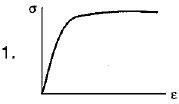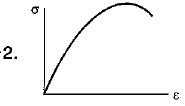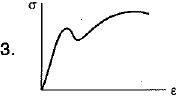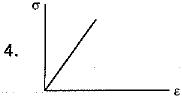Codes:
A B C D
(a) 3 1 4 1
(b) 3 2 4 2
(c) 2 4 3 1
(d) 4 1 3 2

Solution:
QUESTION: 24

Match List-I (Types of tests and Materials) with List-ll (Types of Fractures) and select the correct answer using the codes given below the lists:.
List-I
A. Tensile test on Cl
B. Torsion test on MS
C. Tensile test on MS
D. Torsion test on Cl
List-II
1. Plain fracture on a transverse plane
2. Granular helicoidal fracture
3. Plain granular at 45° to the axis
4. Cup and cone
5. Granular fracture on a transverse plane
Codes:
A B C D
(a) 4 2 3 1
(b) 5 1 4 2
(c) 4 1 3 2
(d) 5 2 4 1

Solution:

In Tension test of ductile materials like mild steel, cup and cone failure occurs and failure plane is at 45° from the longitudinal axis. Where as in tensile test of brittle materials like cast iron, granular fracture occurs and failure plane is at 90° with longitudinal axis.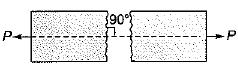In Torsion test of brittle material, granular helicoidal fracture takes place and fracture plane is 45° from the longitudinal axis while in case of ductile material, plane fracture on a transverse plane takes place.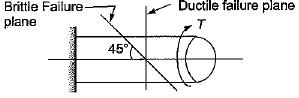QUESTION: 25

Which one of the following properties cannot be evaluated by static tension test?

Solution:
QUESTION: 26

The percentage elongation of a material as obtained from static tension test depends upon the

Solution:

Percentage elongation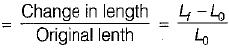where
Lo = original gauge length
L= final length between the gauge mark measured after fracture
since local yielding occurs before the fracture of the specimen, the percentage elongation depends upon the gauge length of the specimen.

QUESTION: 27

Modulus of resilience is

Solution:
QUESTION: 28

Modular ratio of two materials is the ratio of

Solution:
QUESTION: 29

Proof resilience per unit volume of a material is known as .

Solution:
QUESTION: 30

An axial residual compressive stress due to a manufacturing process is present on the outer surface of a rotating shaft subjected to bending. Under a given bending load, the fatigue life of the shaft in the presence of the residual compressive stress is

Solution: